Test: Square Root And Cube Root- 2

# Test: Square Root And Cube Root- 2

Test Description

## 10 Questions MCQ Test UPSC Prelims Paper 2 CSAT - Quant, Verbal & Decision Making | Test: Square Root And Cube Root- 2

Test: Square Root And Cube Root- 2 for Quant 2023 is part of UPSC Prelims Paper 2 CSAT - Quant, Verbal & Decision Making preparation. The Test: Square Root And Cube Root- 2 questions and answers have been prepared according to the Quant exam syllabus.The Test: Square Root And Cube Root- 2 MCQs are made for Quant 2023 Exam. Find important definitions, questions, notes, meanings, examples, exercises, MCQs and online tests for Test: Square Root And Cube Root- 2 below.
Solutions of Test: Square Root And Cube Root- 2 questions in English are available as part of our UPSC Prelims Paper 2 CSAT - Quant, Verbal & Decision Making for Quant & Test: Square Root And Cube Root- 2 solutions in Hindi for UPSC Prelims Paper 2 CSAT - Quant, Verbal & Decision Making course. Download more important topics, notes, lectures and mock test series for Quant Exam by signing up for free. Attempt Test: Square Root And Cube Root- 2 | 10 questions in 10 minutes | Mock test for Quant preparation | Free important questions MCQ to study UPSC Prelims Paper 2 CSAT - Quant, Verbal & Decision Making for Quant Exam | Download free PDF with solutions
 1 Crore+ students have signed up on EduRev. Have you?
Test: Square Root And Cube Root- 2 - Question 1

### How many two-digit numbers satisfy this property : The last digit (units digit) of the square of the two-digit number is 8 ?

Detailed Solution for Test: Square Root And Cube Root- 2 - Question 1

A number ending in 8 can never be a perfect square.
12 = 1
22 = 4
32 = 9
42 = 16
52 = 25
62 = 36
72 = 49
82 = 64
92 = 81

Test: Square Root And Cube Root- 2 - Question 2

### if a = 0.2917, then the value of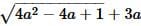is :

Detailed Solution for Test: Square Root And Cube Root- 2 - Question 2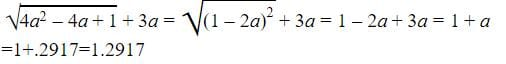Test: Square Root And Cube Root- 2 - Question 3

### A group of students decided to collect as many paise from each member of group as is the number of members. If the total collection amounts to Rs. 59.29, the number of the member is the group is:

Detailed Solution for Test: Square Root And Cube Root- 2 - Question 3

Money collected = (59.29 x 100) paise = 5929 paise.
∴ Number of members = √5929 = 77.

Test: Square Root And Cube Root- 2 - Question 4

if √7 = 2.645, then find the value of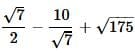Detailed Solution for Test: Square Root And Cube Root- 2 - Question 4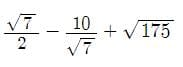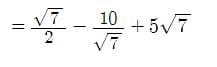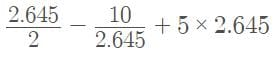= 1.3225 - 3.78071 + 13.225
= 10.76679 ≈ 10.77

Test: Square Root And Cube Root- 2 - Question 5

What is smallest number with which 5400 may be multiplied so that the product is perfect cube?

Detailed Solution for Test: Square Root And Cube Root- 2 - Question 5

Find prime factors of 5400

5400=2×3×3×3×2×2×5×5

If we group them in the group of 3

5400=(2×2×2)×(3×3×3)×5×5

Here to make group of 3 for 5

We have to multiply 5400 by 5.

Test: Square Root And Cube Root- 2 - Question 6

The square root of (14 + 2√13)(14 - 2√13) is

Detailed Solution for Test: Square Root And Cube Root- 2 - Question 6

As we know that  (a²-b²) = (a+b) (a-b)
Therefore 14² - 2²x13 = 144.
So √144 = 12.

Test: Square Root And Cube Root- 2 - Question 7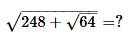Detailed Solution for Test: Square Root And Cube Root- 2 - Question 7

= [√248+√64]
= √248+8
= √256
= 16

Test: Square Root And Cube Root- 2 - Question 8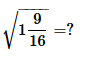Detailed Solution for Test: Square Root And Cube Root- 2 - Question 8

√1 9/16
= √25/16
= 5/4
= 1 1/4.

Test: Square Root And Cube Root- 2 - Question 9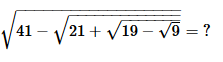Detailed Solution for Test: Square Root And Cube Root- 2 - Question 9

√41-√21+√19-√9
= √41-√21+√19-3
=√41-√21+√16
=√41-√21+4
=√41-√25
=√41-5
=√36
=6

Test: Square Root And Cube Root- 2 - Question 10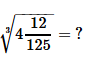Detailed Solution for Test: Square Root And Cube Root- 2 - Question 10

Cube root of (512)/125
= 8/5
Convert it into mixed fraction, we get
= 1 3/5

## UPSC Prelims Paper 2 CSAT - Quant, Verbal & Decision Making

67 videos|50 docs|151 tests
 Use Code STAYHOME200 and get INR 200 additional OFF Use Coupon Code
Information about Test: Square Root And Cube Root- 2 Page
In this test you can find the Exam questions for Test: Square Root And Cube Root- 2 solved & explained in the simplest way possible. Besides giving Questions and answers for Test: Square Root And Cube Root- 2, EduRev gives you an ample number of Online tests for practice

## UPSC Prelims Paper 2 CSAT - Quant, Verbal & Decision Making

67 videos|50 docs|151 tests